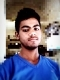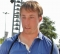## Breadth First Search (BFS) for a GraphUpdated on 16-Jun-2020 11:03:00
The Breadth First Search (BFS) traversal is an algorithm, which is used to visit all of the nodes of a given graph. In this traversal algorithm one node is selected and then all of the adjacent nodes are visited one by one. After completing all of the adjacent vertices, it moves further to check another vertex and checks its adjacent vertices again.To implement this algorithm, we need to use the Queue data structure. All the adjacent vertices are added into the queue when all adjacent vertices are completed, one item is removed from the queue and start traversing through that ... Read More

## Biconnected GraphUpdated on 16-Jun-2020 09:20:10
An undirected graph is said to be a biconnected graph, if there are two vertex-disjoint paths between any two vertices are present. In other words, we can say that there is a cycle between any two vertices.We can say that a graph G is a bi-connected graph if it is connected, and there are no articulation points or cut vertex are present in the graph.To solve this problem, we will use the DFS traversal. Using DFS, we will try to find if there is any articulation point is present or not. We also check whether all vertices are visited by ... Read More

## Median of two sorted arrayUpdated on 16-Jun-2020 09:23:44
Medians are the middle numbers, in other words, the median value is the middle observation in an ordered list. It corresponds to the cumulative percentage of 50%.The size of two arrays must be same, we will find the median of two separate arrays at first, then compare the separate medians to get an actual median of two lists.Input and OutputInput: Two sorted array are given. Array 1: {1, 2, 3, 6, 7} Array 2: {4, 6, 8, 10, 11} Output: The median from two array. Here the median value is 6. Merge the given lists into one. {1, 2, 3, ... Read More

## Count Inversions in an arrayUpdated on 16-Jun-2020 09:29:16
The inversions of an array indicate; how many changes are required to convert the array into its sorted form. When an array is already sorted, it needs 0 inversions, and in another case, the number of inversions will be maximum, if the array is reversed.To solve this problem, we will follow the Merge sort approach to reduce the time complexity, and make it in Divide and Conquer algorithm.Input and OutputInput: A sequence of numbers. (1, 5, 6, 4, 20). Output: The number of inversions required to arrange the numbers into ascending order. Here the number of inversions are 2. First ... Read More

## Peak Element in 2D arrayUpdated on 16-Jun-2020 09:33:16
An item is said to be a peak element when it is greater than or equal with all four neighbor of that element. The neighbor elements are the top, bottom, left and right elements. For this problem, we will consider some bounds. The diagonal elements are not checked as neighbor elements. More than one peak element may present in a matrix, and the peak element is not necessarily the largest element in the matrix.Input and OutputInput: A matrix of different numbers. 10  8  10  10 14 13  12  11 15  9  11  11 15  9  11  21 16 17  19 ... Read More

## Closest Pair of Points ProblemUpdated on 16-Jun-2020 09:37:05
In this problem, a set of n points are given on the 2D plane. In this problem, we have to find the pair of points, whose distance is minimum.To solve this problem, we have to divide points into two halves, after that smallest distance between two points is calculated in a recursive way. Using distances from the middle line, the points are separated into some strips. We will find the smallest distance from the strip array. At first two lists are created with data points, one list will hold points which are sorted on x values, another will hold data ... Read More

## Max Number By SwappingUpdated on 16-Jun-2020 09:39:27
In this problem, one positive integer string is given, we have to find the permutation whose value is maximum by swapping digits’ k times, into different places.We will solve this problem by choosing a digit and swap it with digits following it one at a time to find a maximum number. We repeat the process k times. The backtracking strategy is working here because when we find a number which is not greater than the previous value, we backtrack to old value and check again.Input and OutputInput: A number of multiple digits. The input is: 129814999 Output: The maximum value ... Read More

## Word Break ProblemUpdated on 16-Jun-2020 09:42:44
In the input of this problem, one sentence is given without spaces, another dictionary is also provided of some valid English words. We have to find the possible ways to break the sentence in individual dictionary words.We will try to search from the left of the string to find a valid word when a valid word is found, we will search for words in the next part of that string.Input and OutputInput: A set of valid words as dictionary, and a string where different words are placed without spaces. Dictionary: {mobile, sam, sung, man, mango, icecream, and, go, i, love, ... Read More

## Tug of War AlgorithmUpdated on 16-Jun-2020 09:44:58
In this problem a set of integers are given, we have to break them into two parts, such that the difference of the sum of two subsets is minimum as possible. So our target is to divide two groups of nearly equal strength to participate in the Tug of war game.If the size of subset n is even, it must be divided into n/2, but for the odd value of n, then the size of one subset must be (n-1)/2, and size of another subset must be (n+1)/2.Input and OutputInput: A set of different weights. {23, 45, -34, 12, 0, ... Read More

## The Knight’s tour problemUpdated on 16-Jun-2020 09:51:09
In chess, we know that the knight can jump in a special manner. It can move either two squares horizontally and one square vertically or two squares vertically and one square horizontally in each direction, So the complete movement looks like English letter ‘L’.In this problem, there is an empty chess board, and a knight starting from any location in the board, our task is to check whether the knight can visit all of the squares in the board or not. When It can visit all of the squares, then place the number of jumps needed to reach that location ... Read More